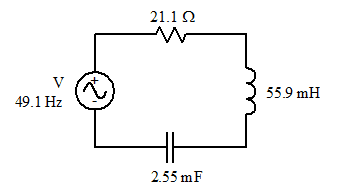# Change in Capacitor for largest possible Current

• Jess_18033152

## Homework Statement

The capacitor is changed so that the largest possible current is supplied by the source.
Enter the value of the capacitive reactance when this change is made.

## Homework Equations

The circuit is powered by an alternating voltage source, which produces a voltage that varies sinusoidally and has an RMS voltage of V.

- The frequency of the voltage source is 49.1 Hz and the inductor has an inductance of 55.9 mH.
Calculate the inductive reactance (XL) of the inductor.

- The capacitor has a capacitance of 2.55 mF.
Calculate the capacitive reactance (XC) of the capacitor. The frequency is still 49.1 Hz.

- The resistor has a resistance of 21.1 Ω.
Calculate the impedance (Z) of the RLC network.

Im not sure what this question is asking me or what equation to use to calculate it either.

What is the sign of the capacitive reactance and inductive reactance?

How are your components interconnected? Is it a series RLC circuit like this?